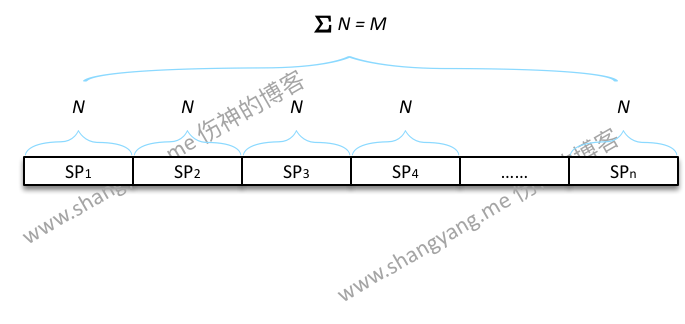➭ 试验 10 次

➭ 试验 100 次

➭ 试验 1000 次

➭ 试验 10000 次

➭ 无限多次

➭ 结论

# 排列和组合计算的到底是什么？

1. 假设有编号为 1、2、3、4、5 的五个小球，试问从中随机抽取 1 个小球，试问每个等可能的样本点被抽取的(等)概率既 $p_1$ 是多少？
因为随机抽取 1 个小球，所以一个样本点由 1 个小球组成；那么现在要求解的是，该事件的样本空间 $\Omega$ 是多少？因为抽取动作只有 1 个步骤，所以根据乘法原理，从 5 个球中抽取 1 个球的所有途径之和 $= 5$，这就是要求的样本空间，又假设，样本空间中所有样本点的概率之和 $= 1$，那么有 $$p_1 = \frac{1}{5} = 0.2$$，所以，该事件中，每个小球可被抽取的(等)概率是 0.2；
2. 假设有编号为 1、2、3、4、5 的五个小球，试问从中随机抽取 2 个小球，试问每个等可能的样本点被抽取的(等)概率 $p_2$ 是多少？
因为随机抽取 2 个小球，所以一个样本点由 2 个小球组成，那么两个小球同时被抽中的可能性是多少呢？ 要求解这个问题，首先要问的是，这是不是重复排列的问题，笔者打算对这两种情况分别求解，

重复排列
如果是有放回的进行抽样，自然这就是一个重复排列的问题，所以整个样本空间的样本点的总数 $= 5 \times 5 = 25$，假设样本空间中所有样本点的概率之和 $= 1$，那么 $$p_2 = \frac{1}{25} = 0.04$$

排列

如果是不放回的进行抽样，自然这就是一个排列的问题，所以整个样本空间的样本点的总数 $= 5 \times 4 = 20$，假设样本空间中所有样本点的概率之和 $= 1$，那么 $$p_2 = \frac{1}{20} = 0.05$$

3. 假设有编号为 1、2、3、4、5 的五个小球，其中 3、5 为不合格品，试问从中随机不放回的抽取 3 个小球，试问恰好抽中 2 个不合格品的样本点概率 $p_3$ 是多少（考虑先后出现的顺序）？恰好抽中 2 个不合格品事件的概率 $p_4$ 是多少？
第一个问题很好求解，因为即便是包含 2 个不合格品的样本点在整个样本空间中与其它样本点而言都是等可能的，所以，$$p_3 = \frac{1}{P^5_3} = \frac{1}{60} = 0.01667$$
第二个问题就非常的有意思了，问的是包含 2 个不合格品的该事件的概率是多少？首先，我们知道，一个事件就是由与之相关的所有的样本点所组成的集合，假设该事件记为事件 $A$，那么事件 $A$ 所包含的所有样本点的(等)概率之和可以通过如下的方式求得，
$$p_4 = P^3_1 \times P^2_1 \times \binom{2}{1} \times p_3 = 2 \times 3 \times 2 \times 0.01667 = 0.2 \dots 求解公式\phantom{1}①$$

备注，思路是，在已抽取到两个不合格产品的情况下，总共的排列有多少种可能；

如果之前有学过概率的同学如果是通过对公式死记硬背的方式来进行学习的，那么看到上面这个求解的公式一定会很惊讶，这个与书本上的求解公式完全不同呀，并且压根没有这种推算的方式；其实上面的这个笔者所给出的公式才是触及到了概率学所要探求的核心，既是求解该事件中所有样本点的等概率之和，因此它就是事件 $A$ 的概率；回过头来，让我们再看看教材中的解法，
$$p_4 = \frac{P^3_1 \times P^2_1 \times \binom{2}{1}}{P^5_3} = \frac{12}{60} = 0.2 \dots 求解公式\phantom{1}②$$
可见，两者的结果出奇的吻合，细心的读者可能会发现，这两个公式本质就是同一个方程，因为 $p_3 = \frac{1}{P^5_3}$，由此我们有，
$$p_4 = P^3_1 \times P^2_1 \times \binom{2}{1} \times p_3 = P^3_1 \times P^2_1 \times \binom{2}{1} \times \frac{1}{P^5_3} = \frac{P^3_1 \times P^2_1 \times \binom{2}{1}}{P^5_3}$$
虽然两个公式本质上求解的是同一个方程，但是描述的事务的方式却完全不同，而教科书中所使用的公式 ② 笔者并不推荐大家在学习概率学的时候，用这个方程去理解概率学本身，因为它省略了非常重要的一个因素，那就是等概率 $p$，将公式 ② 写完整了，实际上应该是
$$p_4 = \frac{P^3_1 \times P^2_1 \times \binom{2}{1} \times p_3}{P^5_3 \times p_3} = \frac{12 \times p_3}{60 \times p_3} = 0.2 \dots 求解公式\phantom{1}②$$
这样，我们就非常的清楚了，实际上概率所重点关注就是某个事件的概率，而该事件的概率实际上就是由该事件的所有样本点的(等)概率之和与样本空间中所有样本点的(等)概率之和的比值，当然也可以像公式 ① 那样先求得每个等可能样本点的概率大小，然后乘以该事件的所有样本点总数的方式来求解；最后笔者所要提及的是，公式 ① 才是概率学的真正本质所在；

# 等概率样本点